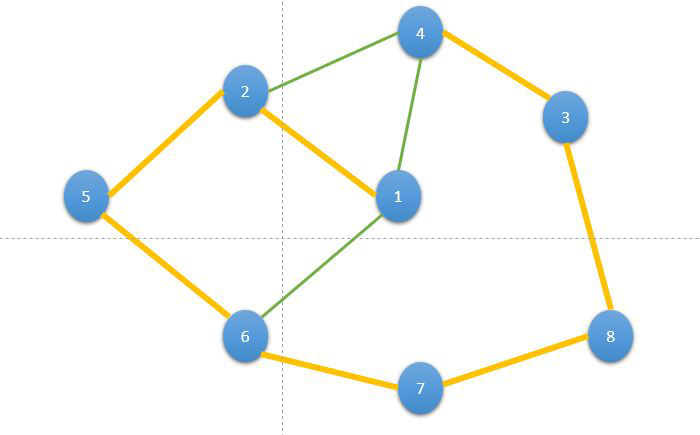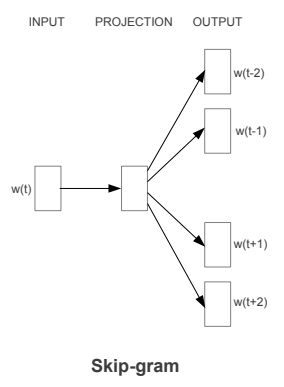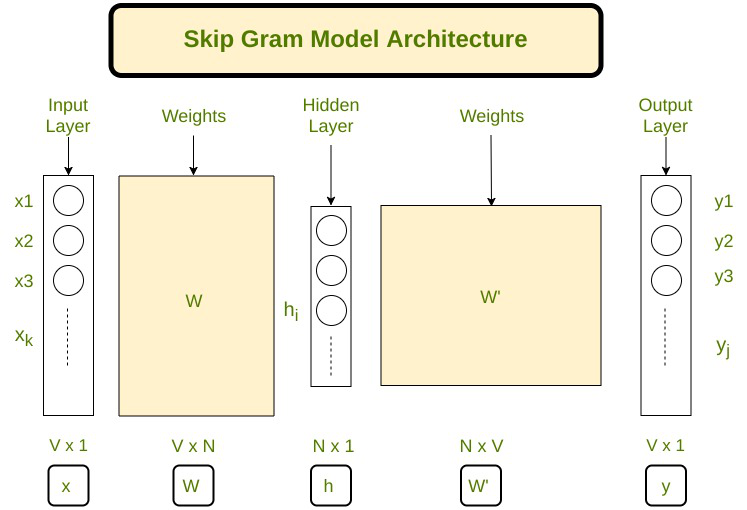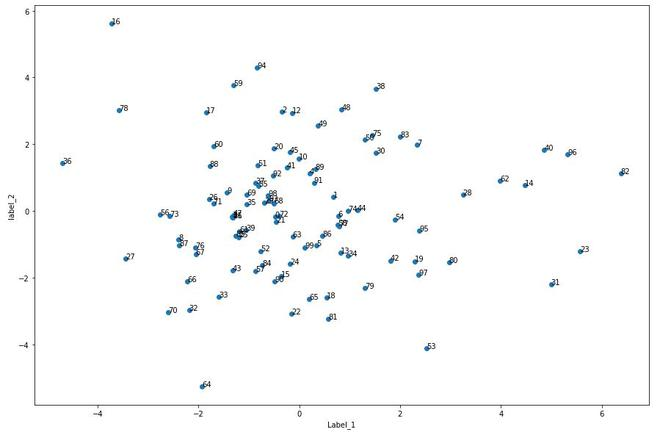Related Articles

# DeepWalk Algorithm

• Last Updated : 18 Jul, 2021

### Graph Data Structure:

In the real world, Networks are just the collection of interconnected nodes. To represent this type of network we need a data structure that is similar to it. Fortunately, we have a data structure that is the graph.

The graph contains vertices (which represents the node in the network) that are connected by edges (which can represent interconnection b/w nodes)

### Deep Walk:

The deep walk is an algorithm proposed for learning latent representations of vertices in a network. These latent representations are used to represent the social representation b/w two graphs. It uses a randomized path traversing technique to provide insights into localized structures within networks. It does so by utilizing these random paths as sequences, that are then used to train a Skip-Gram Language Model.

Skip-Gram Model is used to predict the next word in the sentence by maximizing the co-occurrence probability among the words that appear within a window, w, in a sentence. For our implementation, we will use the Word2Vec implementation which uses the cosine distance to calculate the probability.

Deepwalk process operates in few steps:

• The random walk generator takes a graph G and samples uniformly a random vertex vi as the root of the random walk Wvi. A walk sample uniformly from the neighbors of the last vertex visited until the maximum length (t) is reached.
• Skip-gram model iterates over all possible collocations in a random walk that appear within the window w. For each, we map each vertex vj to its current representation vector Φ(vj ) ∈ Rd.
• Given the representation of vj, we would like to maximize the probability of its neighbors in the walk (line 3). We can learn such posterior distribution using several choices of classifiers

#### Random Walk

Given an undirected graph G = (V, E), with n =| V | and m =| E |, a natural random walk is a stochastic process that starts from a given vertex, and then selects one of its neighbors uniformly at random to visit.• In the above graph, from 1 we have two nodes 2 and 4. From that, we choose any of them, let’s select 2.
• Now, from 2, we have two choices 4 and 5, we randomly select 5 from them. So our random walk becomes Node 1 → 2 → 5.
• Repeat the above process again and again until we cover all the nodes in the graph. This process is known as a random walk. One such random walk is 1 → 2 → 5 → 6 → 7 → 8 → 3 → 4.

#### Skip-Gram Model

Word Embeddings is a way to map words into a feature vector of a fixed size to make the processing of words easier. In 2013, Google proposed word2vec, a group of related models that are used to produce word embeddings. In the skip-gram architecture of word2vec, the input is the center word and the predictions are the context words. Consider an array of words W, if W(i) is the input (center word), then W(i-2), W(i-1), W(i+1), and W(i+2) are the context words if the sliding window size is 2.Below is the template architecture for the skip-gram model:• Deepwalk is scalable since it does process the entire graph at once. This allows deep walk to create meaningful representations of large graphs that may not run on the spectral algorithms.
• Deepwalk is faster compared to the other algorithms while dealing with sparsity.
• Deepwalk can be used for many purposes such as Anomaly Detection, Clustering, Link Prediction, etc.

### Implementation

• In this implementation, we will be using networkx and karateclub API. For our purpose, we will use Graph Embedding with Self Clustering: Facebook dataset. The dataset can be downloaded from here

## Python3

 `# Install karateclub API``! pip install karateclub``# imports`` ` `import` `networkx as nx``import` `pandas as pd``from` `sklearn.decomposition ``import` `PCA``import` `matplotlib.pyplot as plt``from` `karateclub ``import` `DeepWalk`` ` `# import dataset``df ``=` `pd.read_csv(``"/content/politician_edges.csv"``)``df.head()`` ` `# create Graph``G ``=` `nx.from_pandas_edgelist(df, ``"node_1"``, ``"node_2"``, create_using``=``nx.Graph())``print``(``len``(G))`` ` `# train model and generate embedding``model ``=` `DeepWalk(walk_length``=``100``, dimensions``=``64``, window_size``=``5``)``model.fit(G)``embedding ``=` `model.get_embedding()`` ` `# print Embedding shape``print``(embedding.shape)``# take first 100 nodes``nodes ``=``list``(``range``(``100``))`` ` `# plot nodes graph``def` `plot_nodes(node_no):``    ``X ``=` `embedding[node_no]``     ` `    ``pca ``=` `PCA(n_components``=``2``)``    ``pca_out``=` `pca.fit_transform(X)``     ` `     ` `    ``plt.figure(figsize``=``(``15``,``10``))``    ``plt.scatter(pca_out[:, ``0``], pca_out[:, ``1``])``    ``for` `i, node ``in` `enumerate``(node_no):``        ``plt.annotate(node, (pca_out[i, ``0``], pca_out[i, ``1``]))``    ``plt.xlabel(``'Label_1'``)``    ``plt.ylabel(``'label_2'``)``    ``plt.show()`` ` `plot_nodes(nodes)`

Output:

```node_1    node_2
0    0    1972
1    0    5111
2    0    138
3    0    3053
4    0    1473
-------------
# length of nodes
5908
-------------
# embedding shape
(5908, 64)```Plot of Nodes

References:

Attention reader! Don’t stop learning now. Get hold of all the important Machine Learning Concepts with the Machine Learning Foundation Course at a student-friendly price and become industry ready.

My Personal Notes arrow_drop_up